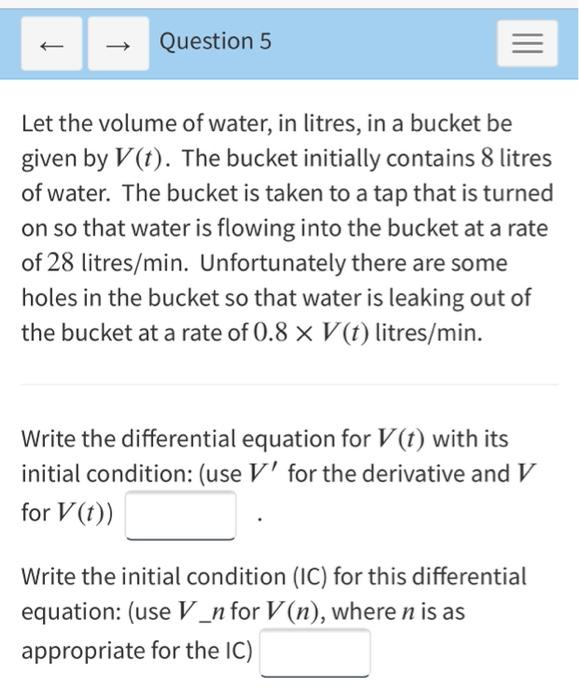### Create an Account

Already have account?

### Forgot Your Password ?

Home / Questions / Question 5 Let the volume of water, in litres, in a bucket be given by V(t). The bucket in...

# Question 5 Let the volume of water, in litres, in a bucket be given by V(t). The bucket initially contains 8 litres of water. The bucket is taken to a tap that is turned on so that water is flowing in

Question 5 Let the volume of water, in litres, in a bucket be given by V(t). The bucket initially contains 8 litres of water. The bucket is taken to a tap that is turned on so that water is flowing into the bucket at a rate of 28 litres/min. Unfortunately there are some holes in the bucket so that water is leaking out of the bucket at a rate of 0.8 x V(t) litres/min. Write the differential equation for V(t) with its initial condition: (use V' for the derivative and V for V(t)) Write the initial condition (IC) for this differential equation: (use V_n for V(n), where n is as appropriate for the IC)Apr 14 2021 View more View Less

#### Answer (Solved)Subscribe To Get Solution